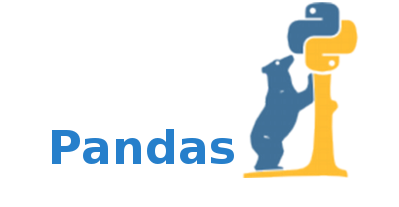## Pandas的秘密：5个鲜为人知的功能，将彻底改变你的数据分析技能

Pandas是一个功能强大的Python库，用于数据操作和分析。数据科学家和分析师一直在使用它来清洗、更改和分析数据。虽然许多人都熟悉Pandas的基本功能，但还有一些不太为人所知的功能可以帮助你更高效地处理数据。在这篇文章中，我们将探索关于Pandas的五个鲜为人知的秘密。

1 数据透视表功能

Pandas中的数据透视表功能是一个汇总和聚合数据的强大工具。它允许你按一列或多列对数据进行分组，并对分组后的数据应用聚合函数。下面是一个如何使用pivot_table函数的例子：

``````import pandas as pd

# load the Titanic dataset from GitHub
url = 'https://raw.githubusercontent.com/pandas-dev/pandas/master/doc/data/titanic.csv'

# create a pivot table that shows the total fares paid by passengers in each passenger class
# who either survived or did not survive
pivot = pd.pivot_table(df, index='Pclass', columns='Survived', values='Fare', aggfunc='sum')

# display the resulting pivot table
pivot``````

2 重塑数据

``````import pandas as pd

# load the iris dataset from GitHub
url = 'https://raw.githubusercontent.com/pycaret/pycaret/master/datasets/iris.csv'

# reshape the data using the melt method to create a long format dataset
melted = pd.melt(df, id_vars=['species'], var_name='measurement', value_name='value')

# display the resulting melted dataset
melted``````

3 自定义聚合函数

Pandas提供了广泛的内置聚合函数，比如sum、mean和count。但是，你可能会遇到需要对数据应用自定义聚合函数的情况。Pandas允许你使用aggmethod定义和应用自定义聚合函数。下面是如何定义和应用自定义聚合函数的示例：

``````# import pandas library
import pandas as pd

# Creating DataFrame
df = pd.DataFrame(
{'stud_id' : [101, 102, 103, 104,
101, 102, 103, 104],
'sub_code' : ['CSE6001', 'CSE6001', 'CSE6001',
'CSE6001', 'CSE6002', 'CSE6002',
'CSE6002', 'CSE6002'],
'marks' : [77, 86, 55, 90,
65, 90, 80, 67]}
)

# Displaying DataFrame
df``````

``````# Importing reduce for
# rolling computations
from functools import reduce

# define a Custom aggregation
# function for finding total
def total(series):
return reduce(lambda x, y: x + y, series)

# Grouping the output according to
# student id and printing the corresponding
# total marks and to check whether the
# output is correct or not, sum function
# is also used to print the sum.
df.groupby('stud_id').agg({'marks': ['sum', total]})``````

4 DateTime功能

Pandas提供了许多用于处理日期和时间数据的函数。这些函数允许你从datetime对象中提取信息，例如星期几或一天中的某一小时。下面是一个如何从datetime对象中提取星期几的例子：

``````import pandas as pd

# load the female births dataset from GitHub
url = 'https://raw.githubusercontent.com/jbrownlee/Datasets/master/daily-total-female-births.csv'

# convert the Date column to datetime format
df['Date'] = pd.to_datetime(df['Date'])

# add a new column 'DayOfWeek' to the DataFrame and populate it with the day name
df['DayOfWeek'] = df['Date'].dt.day_name()

# display the resulting DataFrame
df``````

5 内存优化

``````import pandas as pd

# load the sales dataset from GitHub
url = 'https://raw.githubusercontent.com/jbrownlee/Datasets/master/daily-total-female-births.csv'

# convert the 'Births' column to int32 data type from int64
df['Births'] = df['Births'].astype('int32')

# check new dtypes
df.dtypes``````